Download Presentation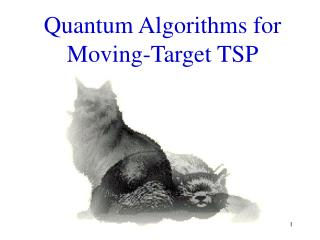Quantum Algorithms for Moving-Target TSP

# Quantum Algorithms for Moving-Target TSP - PowerPoint PPT Presentation

Quantum Algorithms for Moving-Target TSP. Two Slit Experiment. Bullets. Two Slit Experiment. Sound Waves. Two Slit Experiment. Electrons. Two Slit Experiment. Observing Electrons. Basic Quantum Computation. Qubit - can be 1, 0 or both 1 and 0 |x> - number in Quantum ComputerI am the owner, or an agent authorized to act on behalf of the owner, of the copyrighted work described.
Download Presentation## Quantum Algorithms for Moving-Target TSP

An Image/Link below is provided (as is) to download presentation

Download Policy: Content on the Website is provided to you AS IS for your information and personal use and may not be sold / licensed / shared on other websites without getting consent from its author.While downloading, if for some reason you are not able to download a presentation, the publisher may have deleted the file from their server.

- - - - - - - - - - - - - - - - - - - - - - - - - - E N D - - - - - - - - - - - - - - - - - - - - - - - - - -
Presentation Transcript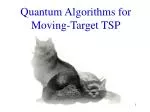### Quantum Algorithms for Moving-Target TSP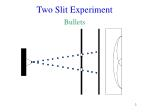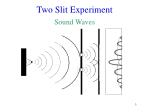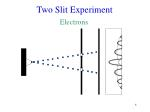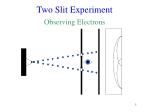Two Slit Experiment

Observing Electrons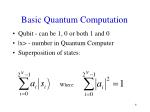Basic Quantum Computation
• Qubit - can be 1, 0 or both 1 and 0
• |x> - number in Quantum Computer
• Superposition of states:

Where: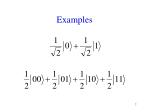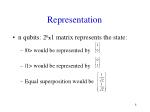Representation
• n qubits: 2nx1 matrix represents the state:
• |0> would be represented by
• |1> would be represented by
• Equal superposition would be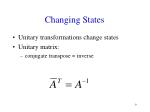Changing States
• Unitary transformations change states
• Unitary matrix:
• conjugate transpose = inverse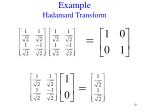Example: CNot Gate

Not Gate:

CNot Gate:Grover’s Search Algorithm
• Search a phone book for a specific number without rearranging the numbers.
• Idea: magnify amplitude of the “choosen” number:
• Flip the amplitude of the selected item and rotate all amplitudes around the average
• Repeat this until the selected item’s probability of being read is greater than 1/2Graphical Representation

Original Amplitudes

Negate Amplitude

Average of all Amplitudes

Flip all Amplitudes around AvgTime Complexity
• Normal search requires N/2 steps
• Grover’s Algorithm takes steps2

3

Origin

1

4

Moving-Target TSP4

6

a

(V=0)

11

12

5

e

2

b

(V=0)

(V=0)

3

8

10

c

d

(V=0)

(V=0)

Moving Target TSP is Intractable
• NP-Hard
• Classical TSP is NP-Complete
• Classical TSP reduces to Moving-Target TSPNP-Complete
• Contained in NP:

1. Decision version: \$ path with time < T?

• Non-deterministically travel all paths. If one exists with time < T, return TRUE. Else, return FALSE

2. Optimization version: what is min-time path?

• Upper-bound T with initial random path. Then, binary search the range by testing T/2, T/4, etc. to find optimal the minimum- time pathQuantum Computing Solution
• Step 1 - Traverse every possible path
• Step 2 - Search through paths superposition to find a shortest pathSuperposition for Hamiltonian Paths
• T. Rudolph
• superposition of cubic bipartite graph in linear time
• Cubic - all nodes have degree 3
• Bipartite
• nodes are partitioned into two groups
• each node is only adjacent to nodes in other groupPotential Problems
• Problem 1:
• Extract the Hamiltonian paths
• Problem 2:
• Find cycles, not paths
• Problem 3:
• Only works for cubic graphsSolution to Problem 1
• How to extract only paths containing all 1’s in first register?
• Solution: Use Grover’s Algorithm!Solution to Problem 2
• Use black box for Hamiltonian paths to solve for Hamiltonian cycles?
• Solution:Solution to Problem 3
• Problem 3: non-cubic graphs
• Solution:
• Make all nodes have the same degree
• Degree must be a power of 2
• Algorithm when all nodes have degree 2iGroups of x nodes

Step 1Give all nodes same degree of 2x
• Graph G has n nodes
• Find node with largest degree D
• Find x where

n+x-n(mod x)G

Step 1 (continued)
• Go through the graph G node by node, and go through the new nodes set by set:H

H

H

H

Algorithm

Control bit

• Quantum transition for nodes with 2x degree:Observations
• Algorithm works for all graphs
• May double # of nodes
• Takes O(n) steps
• Search first register for all 1’s
• Added nodes do not affect solutionExtension to TSP
• Need another register:
• Large enough to hold longest path
• At each step, add edge weight into registerIteration
• All paths of length n & their weights
• Run algorithm for Hamiltonian cycles
• Look for all 1’s in the first register
• Superposition of all Hamiltonian cycles
• Grover’s algorithm on sum register finds minExtension to Moving-Target TSP
• Moving Target TSP
• Each node has a velocity
• Find the minimum-weight round trip

v1y

v2x

v1x

v2yMoving-Target TSP Solution
• Add a time register
• Track the total time elapsed so far
• Each step: calculate time to reach next node

v1y

MaxV

v1xDetermining the Optimal Path
• Add time to reach next node to total sum
• Find the minimum, similar to TSP
• Added time complexity is linearSummary
• Extended Hamiltonian path algorithm for cubic bipartite graphs [Rudolph’s]
• For TSP and Moving-Target TSP, paths superposition can be obtained in linear time
• Grover’s search algorithm works in time SQRT of # of objects in superposition
• 2n different paths: total time is O(2n/2 )Future Work
• Faster algorithm for (Moving-Target) TSP
• Improve Grover’s search algorithm
• P=NP for quantum computation?Circuit

j

i

a

V

A

b

c

a

j+1

b

c

• a,b,c are the nodes adjacent to I
• A is the register keeping track of which nodes
• have been traversedFinding the Minimum
• Measure the lengths register (M)
• Create superposition again
• Now search for all paths < M
• On average, do this log(k) times
• k is the number of items in the superpositionBullets

Sound Waves

Electrons

Observed Electrons